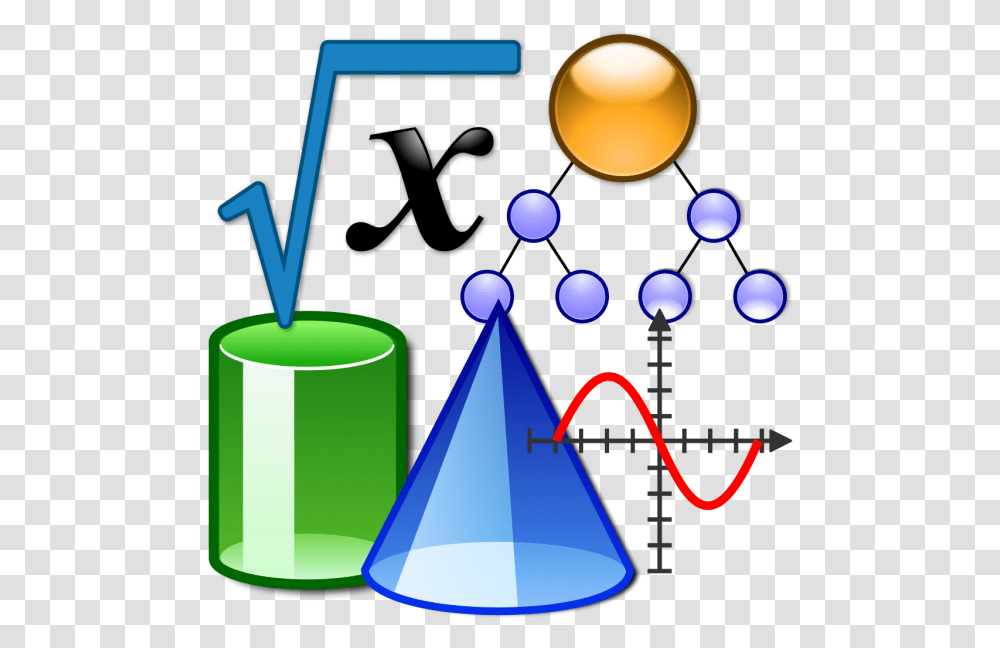# Mathematical Game Mathematical Problem Algebraic Equation High School Math Clipart, Cone Transparent Png0 by B Boomer on 11.06.2020 8 104Free download Mathematical Game Mathematical Problem Algebraic Equation High School Math Clipart, Cone Transparent Png (586x588) for free. All images with the background cleaned and in PNG (Portable Network Graphics) format. Additionally, you can browse for other cliparts from related tags on topics algebraic, cone, equation, game. Available Pngset's online clip art editor before downloading.

Related tags:

CC BY-NC 4.0 Licence

Free for personal use

Share

Title: Mathematical Game Mathematical Problem Algebraic Equation High School Math Clipart, Cone Transparent Png

Format: PNG

Dimensions: 586x588 px

Size: 190KB